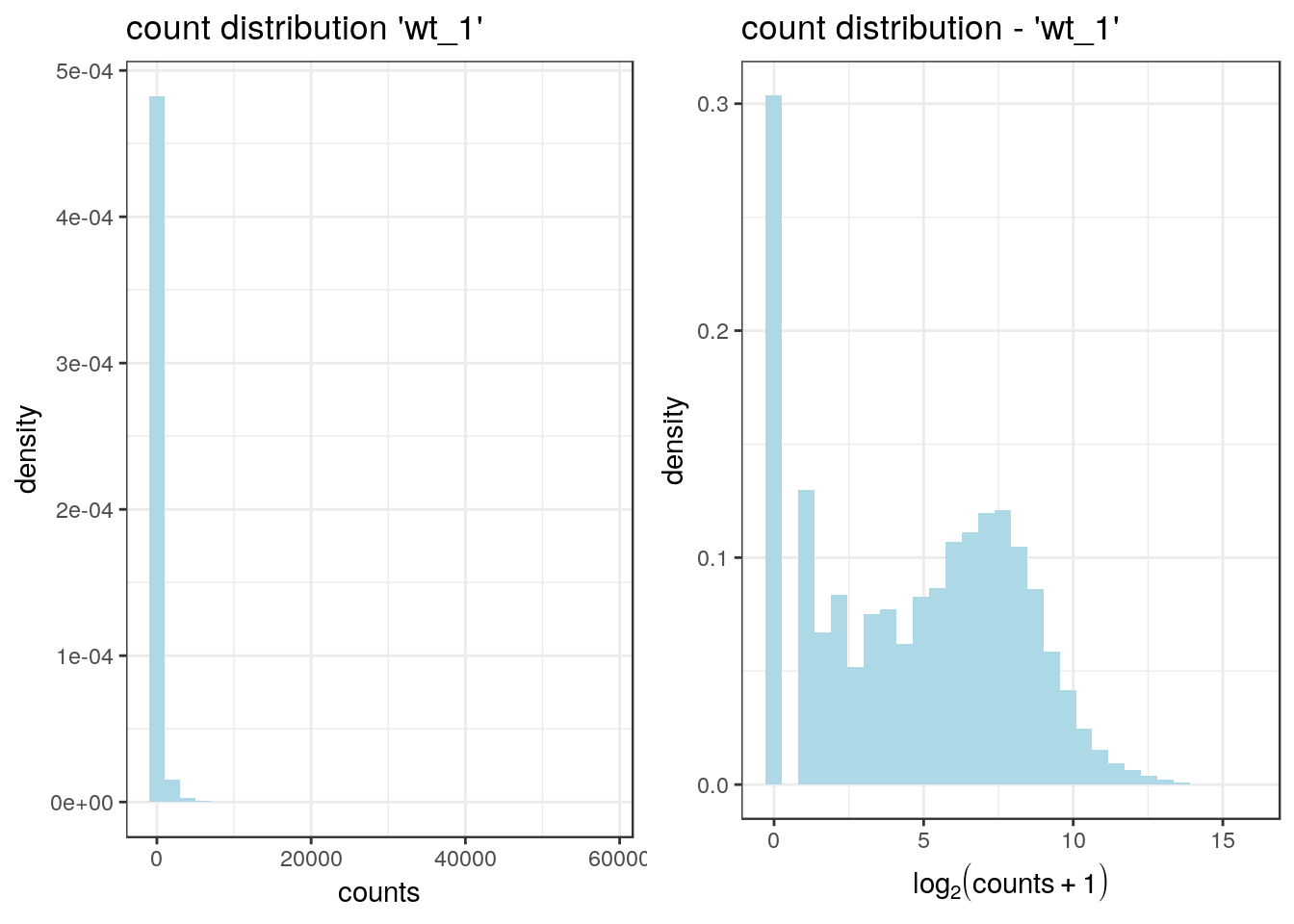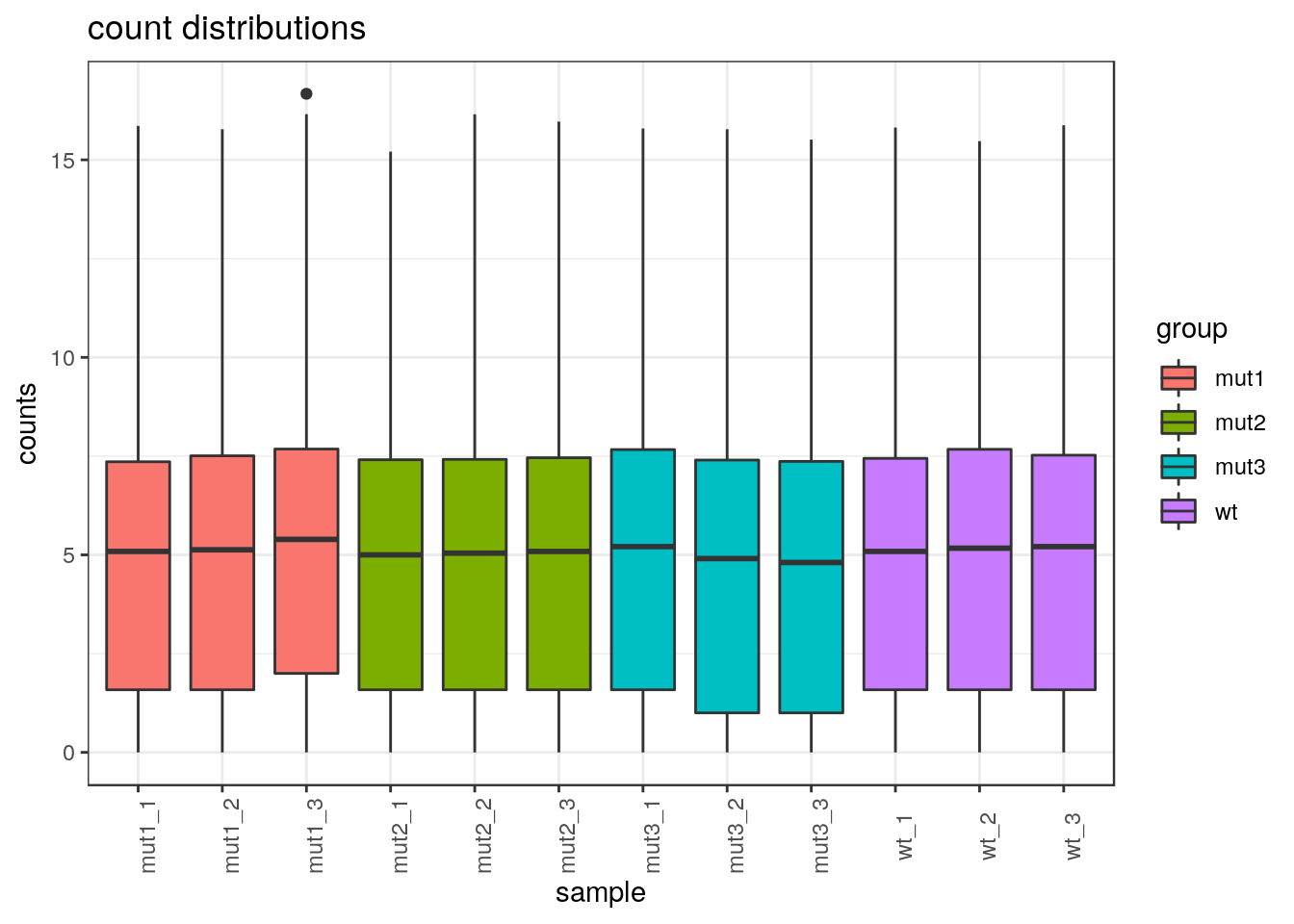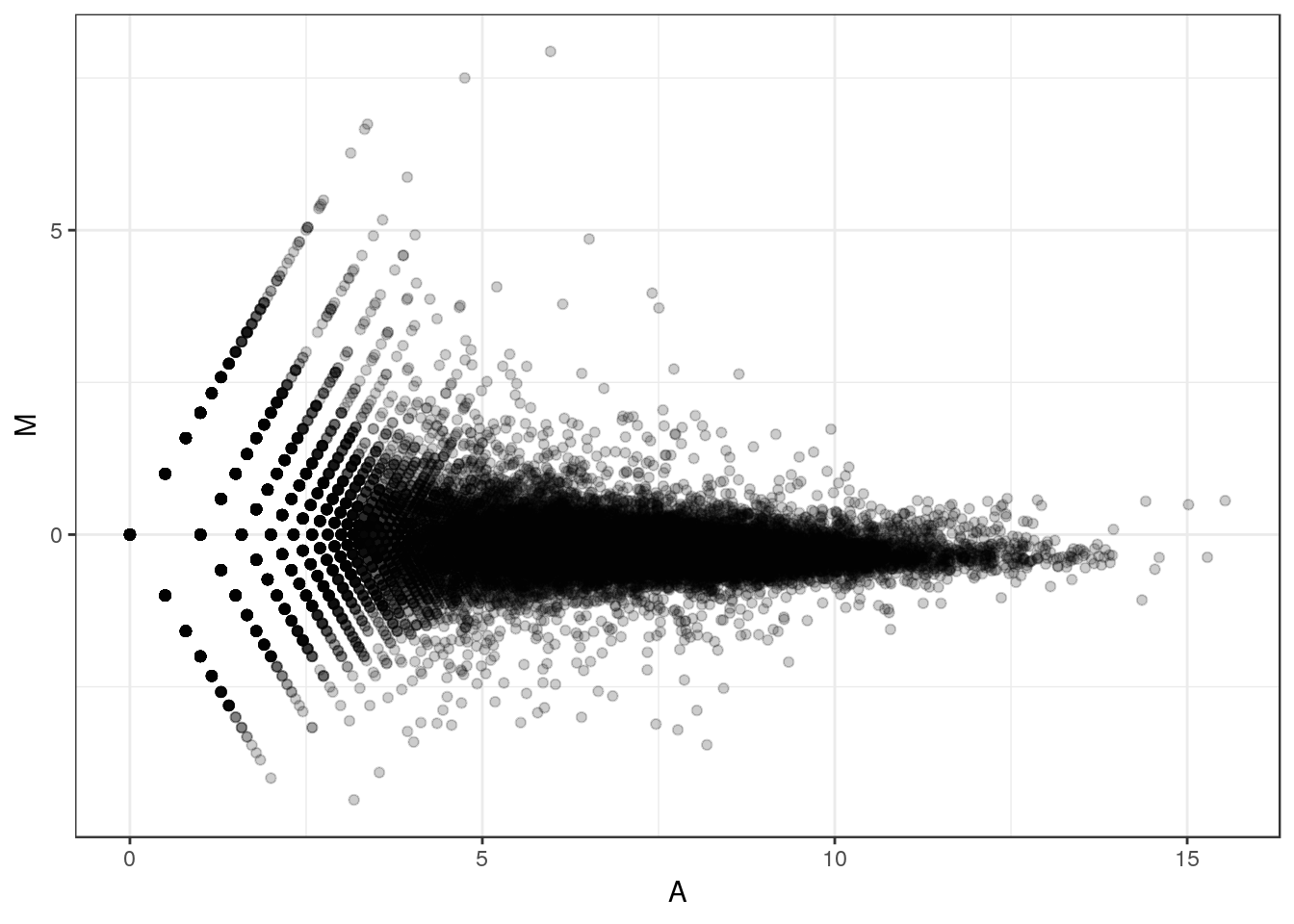This file illustrates the normalization and differential analysis of RNAseq data on a real life dataset. The data are provided by courtesy of the transcriptomic platform of IPS2 and the name of the genes were shuffled. Hence the results are not biologically interpretable. They are composed of three files, all included in the directory `data`:

• `D1-counts.txt` contains the raw counts of the experiment (13 columns: the first one contains the gene names, the others correspond to 12 different samples);

• `D1-genesLength.txt` contains information about gene lengths;

• `D1-targets.txt` contains information about the sample and the experimental design.

In the rest of this file, we:

1/ first import and describe the data;

2/ then perform and compare different normalizations;

3/ finally perform a differential analysis using different methods and models.

We advice that you use the file by reading the R code while trying to make sense of it. Then, you run it and analyze the results. The packages `devtools`, `ggplot2`, `gridExtra`, `reshape2`, `mixOmics`, `RColorBrewer`, `edgeR` and `VennDiagram` are required to compile this file. Information about my system (including package and R versions) are provided at the end of this file (HTML format), in Section “Session information”.

``````library(ggplot2)
library(gridExtra)
library(reshape2)
library(mixOmics)
library(RColorBrewer)
library(edgeR)
library(VennDiagram)
library(devtools)``````

## Data description and importation

The data used in this practical session correspond to 12 samples of RNAseq obtained from microdissections on plants. Plants have four different genotypes: the wild type (“wt”“) plant and three types of mutants and are observed three times in the same conditions. for information Mutants 1 and 2 have a similar phenotype (more complicated leaves than the wild type) whereas mutant 3 has the opposite phenotype (simpler leaves than the wild type).

The datasets are included in the repository `data` located at the root of the material for this practical session. They can be loaded using:

``````raw_counts <- read.table("../data/D1-counts.txt", header = TRUE, row.names = 1)
raw_counts <- as.matrix(raw_counts)
stringsAsFactors = FALSE)
gene_lengths <- scan("../data/D1-genesLength.txt")``````

### Raw counts

The dimensions of the raw count matrix are equal to:

``dim(raw_counts)``
``##  50897    12``

which shows that the data contains 12 columns (samples) and 50897 rows (genes). And a quick look is obtained by:

``head(raw_counts)``
``````##                  wt_1 wt_2 wt_3 mut1_1 mut1_2 mut1_3 mut2_1 mut2_2 mut2_3
## Medtr0001s0010.1    0    0    0      1      0      1      0      0      0
## Medtr0001s0070.1    0    0    0      0      0      0      0      0      0
## Medtr0001s0100.1    0    0    0      0      0      0      0      0      0
## Medtr0001s0120.1    0    0    0      0      0      0      0      0      0
## Medtr0001s0160.1    0    0    0      0      0      0      0      0      0
## Medtr0001s0190.1    0    0    0      0      0      0      0      0      0
##                  mut3_1 mut3_2 mut3_3
## Medtr0001s0010.1      0      1      0
## Medtr0001s0070.1      0      0      0
## Medtr0001s0100.1      0      0      0
## Medtr0001s0120.1      0      0      0
## Medtr0001s0160.1      0      0      0
## Medtr0001s0190.1      0      0      0``````

which shows that the row names are gene names and that the data are made of counts. Many of these counts are equal to 0. A basic exploratory analysis of the count data is provided in the next section.

### Experimental design

The experimental design is described in the object `design`:

``design``
``````##    labels group replicat
## 1    wt_1    wt  repbio1
## 2    wt_2    wt  repbio2
## 3    wt_3    wt  repbio3
## 4  mut1_1  mut1  repbio1
## 5  mut1_2  mut1  repbio2
## 6  mut1_3  mut1  repbio3
## 7  mut2_1  mut2  repbio1
## 8  mut2_2  mut2  repbio2
## 9  mut2_3  mut2  repbio3
## 10 mut3_1  mut3  repbio1
## 11 mut3_2  mut3  repbio2
## 12 mut3_3  mut3  repbio3``````

that contains 3 columns: the first one `labels` is the sample name, the second one `group` is the group of the sample (wild type or one of three types of mutant) and the last one `replicat` is the biological replicate number.

## Basic exploratory analysis of raw counts

We start the analysis by filtering out the genes for which no count has been found:

``````raw_counts_wn <- raw_counts[rowSums(raw_counts) > 0, ]
dim(raw_counts_wn)``````
``##  27916    12``

The number of genes which have been filtered out is thus equal to 22981.

It is often useful, to visualize the count distribution, to compute “pseudo counts”, which are log-transformed counts:

``````pseudo_counts <- log2(raw_counts_wn + 1)
``````##                      wt_1     wt_2     wt_3   mut1_1   mut1_2   mut1_3
## Medtr0001s0010.1 0.000000 0.000000 0.000000 1.000000 0.000000 1.000000
## Medtr0001s0200.1 0.000000 1.000000 0.000000 0.000000 0.000000 0.000000
## Medtr0001s0260.1 6.357552 6.768184 6.643856 6.169925 6.507795 6.189825
## Medtr0001s0360.1 0.000000 0.000000 1.000000 0.000000 0.000000 0.000000
## Medtr0001s0490.1 1.000000 2.000000 0.000000 0.000000 0.000000 0.000000
## Medtr0002s0040.1 7.707359 6.918863 7.826548 2.584963 2.000000 4.643856
##                    mut2_1   mut2_2   mut2_3   mut3_1   mut3_2   mut3_3
## Medtr0001s0010.1 0.000000 0.000000 0.000000 0.000000 1.000000 0.000000
## Medtr0001s0200.1 0.000000 0.000000 0.000000 0.000000 0.000000 0.000000
## Medtr0001s0260.1 6.209453 6.392317 6.672425 6.820179 6.554589 6.539159
## Medtr0001s0360.1 0.000000 1.584963 0.000000 1.000000 0.000000 0.000000
## Medtr0001s0490.1 0.000000 0.000000 1.584963 1.000000 0.000000 1.000000
## Medtr0002s0040.1 6.643856 7.651052 8.044394 3.169925 3.459432 1.584963``````
``````df_raw <- melt(pseudo_counts, id = rownames(raw_counts_wn))
names(df_raw)[1:2]<- c("id", "sample")
df_raw\$method <- rep("Raw counts", nrow(df_raw))
``````##                 id sample    value     method
## 1 Medtr0001s0010.1   wt_1 0.000000 Raw counts
## 2 Medtr0001s0200.1   wt_1 0.000000 Raw counts
## 3 Medtr0001s0260.1   wt_1 6.357552 Raw counts
## 4 Medtr0001s0360.1   wt_1 0.000000 Raw counts
## 5 Medtr0001s0490.1   wt_1 1.000000 Raw counts
## 6 Medtr0002s0040.1   wt_1 7.707359 Raw counts``````

The object `df_raw` will be used later to compare the effect of different normalization on the count distribution in the different samples.

### Count distribution

First, we provide an overview of the distribution of the first sample (without the genes that have been filtered out) by ploting the histograms of raw counts and pseudo counts:

``````df <- data.frame(rcounts = raw_counts_wn[ ,1], prcounts = pseudo_counts[ ,1])

p <- ggplot(data=df, aes(x = rcounts, y = ..density..)) +
geom_histogram(fill = "lightblue") + theme_bw() +
ggtitle(paste0("count distribution '", design\$labels, "'")) +
xlab("counts")

p2 <- ggplot(data=df, aes(x = prcounts, y = ..density..)) +
geom_histogram(fill = "lightblue") + theme_bw() +
ggtitle(paste0("count distribution - '", design\$labels, "'")) +
xlab(expression(log(counts + 1)))

grid.arrange(p, p2, ncol = 2)``````This figure shows that the count distribution is highly skewed with a large proportion of genes with a count equal to 0 and a few number of genes with an extreme count (more that \(2^{15}\)).

An alternative representation can be obtained with boxplots (all samples, colored by group)

``````df <- cbind(design, t(pseudo_counts))
df <- melt(df, id.vars = c("labels", "group", "replicat"))

p <- ggplot(data = df, aes(x = labels, y = value, fill = group)) +
geom_boxplot() + theme_bw() + ggtitle("count distributions") +
xlab("sample") + ylab("counts") +
theme(axis.text.x = element_text(angle = 90))
p``````### Reproducibility between samples (MA plots)

``````df <- data.frame("A" = (pseudo_counts[ ,1] + pseudo_counts[ ,2])/2,
"M" = pseudo_counts[ ,1] - pseudo_counts[ ,2])
p <- ggplot(data = df, aes(x = A, y = M)) + geom_point(alpha = 0.2) + theme_bw()
p``````### Relation between mean and variance

Another important feature of RNAseq data is the fact that they are overdispersed. This means that the variance of the count of a given gene over different biological samples within a given condition is larger than the average count for the same gene. This feature is illustrated by plotting the graphics of the mean vs variance for condition “wt” for all genes with an average count smaller than 5000 (otherwise the chart can not be read easily):

``````df <- data.frame(mean = apply(raw_counts_wn[ ,design\$group == "wt"], 1, mean),
var = apply(raw_counts_wn[ ,design\$group == "wt"], 1, var))
df <- df[df\$mean <= 5000, ]
p <- ggplot(data=df, aes(x = mean, y = var)) + geom_point(colour = "orange") +
theme_bw() + geom_abline(aes(intercept=0, slope=1)) +
ggtitle("Variance versus mean in counts") + ylab("variance")
print(p)``````